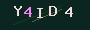# emlog文章缩略图修改，等比例缩放剪切，保持美观

emlog是自带有生成缩略图功能的，不过生成的缩略图特别丑，变形了。。。

1、先看原始的，在include/lib/function.base.php文件中，大约568行，resizeImage方法。
在这个方法中用到了chImageSize，官方注释：按比例计算图片缩放尺寸。。。。。
主要就是这个方法问题。

2、附上修改后的chImageSize方法

`function chImageSize(\$img, \$max_w, \$max_h) {    \$size = @getimagesize(\$img);    \$w = \$size;    \$h = \$size;    //计算缩放比例    @\$w_ratio = \$max_w / \$w;    @\$h_ratio = \$max_h / \$h;	\$ratio = (\$w_ratio < \$h_ratio) ? \$h_ratio : \$w_ratio;	\$ratio = !empty(\$ratio) ? \$ratio : 1;    //决定处理后的图片宽和高    if ((\$w <= \$max_w) && (\$h <= \$max_h)) {        \$tn['w'] = \$w;        \$tn['h'] = \$h;    }else{	\$tn['w'] = ceil(\$max_w / \$ratio);        \$tn['h'] = ceil(\$max_h / \$ratio);	}    \$tn['rc_w'] = \$w;    \$tn['rc_h'] = \$h;    return \$tn;}`

3、在修改一下resizeImage方法
return那一行换掉，换成这个

`return imageCropAndResize(\$img, \$thum_path, 0, 0, 0, 0, \$max_w, \$max_h, \$newwidth, \$newheight);`

### 欢迎 你 来评论1、通过本站购买模板/插件/源码，均享有永久售后服务（仅软件现有功能，不包含开发新增）

2、文章中都有说明教程，在使用过程中遇到问题可以咨询博主。

3、静态HTML页面模板可转换成CMS模板，服务费用100元一页（总费用可协商）。

4、本站的静态HTML页面模板凡超过400元的可免费转换CMS模板一次。

5、购买源码类提供一次免费安装服务（需提供服务器或主机信息）。

6、模板/插件/源码二次打开，此服务按量收费，如有需要，请先和博主商谈报价。

7、自定义模板服务每页100元，如需多终端兼容，每页150元。

8、定制插件服务，300元起（具体看功能需求）。

9、定制系统服务，请整理需求文档和预算，价格具体和博主协商。

10、若有其他问题的网友可联系博主沟通，博主会的一定教（如果问题很复杂，请详谈劳务费哈）。

• QQ
• 二维码支付宝赞助打赏微信赞助打赏